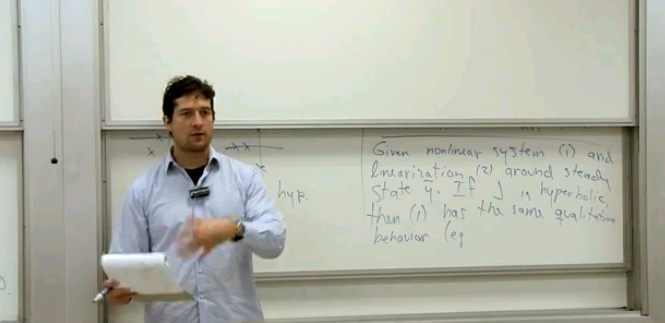# Home

## Math 113B. Lec. 10. Introduction to Mathematical Modeling in Biology: Phase Diagrams III (English)

Recorded: January 29, 2014

Lecture 10: Phase Diagrams III

Course Description: This course is intended for both mathematics and biology undergrads with a basic mathematics background, and consists of an introduction to modeling biological problems using continuous ODE methods (rather than discrete methods as used in 113A). We describe the basic qualitative behavior of dynamical systems in the context of a simple population model and, as time allows, introduce other types of models such as chemical reactions inside the cell or excitable systems leading to oscillations and neuronal signals. Certain topics from linear algebra that are needed for this course are presented as well, so a linear algebra prerequisite is not necessary.